## The Swiss-Knife polynomials.The Swiss-Knife polynomials are closely related to the family of Euler-Bernoulli polynomials and numbers. The coefficients of the polynomials are integers, in contrast to the coefficients of the Euler and Bernoulli polynomials, which are rational numbers (see HMF, page 809). Thus the Swiss-Knife polynomials can be computed more easily and applied to special number theory.

The Euler, Bernoulli, Genocchi, Euler zeta, tangent as well as the up-down numbers and the Springer numbers are either values or scaled values of these polynomials. The Swiss-Knife polynomials are also connected with the Riemann and Hurwitz zeta function. They display a strong and beautiful sinusoidal behavior if properly scaled which has its basis in the Fourier analysis of the generalized zeta function.

The Swiss-Knife polynomials were discovered by Peter Luschny on March 2008. They are possibly new. In fact, references to these polynomials were deleted from Wikipedia (2008-12-27) on the ground that some Wikipedia editors 'strongly suggest that the subject of the article is original research'. If you know an earlier mention in the literature please let me know. (Replace 'www.' by 'peter(at)' in the above URL.)

## The Swiss-Knife Polynomials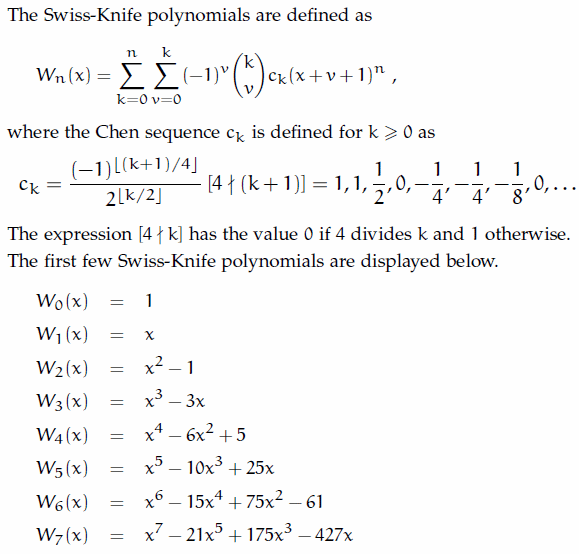## Representations of classical numbersMore on this can be found here: Decompositions of the Euler, Bernoulli, Genocchi, Springer and tangent numbers.

## The sinusoidal character of the polynomials

The scaled Swiss-Knife polynomials are defined asPlotting ωn(x) shows the sinusoidal behavior of these polynomials, which is easily overlooked in the non-scaled form. For odd index ωn(x) approximates sin(xΠ/2) and for even index cos(xΠ/2) in an interval enclosing the origin. This observation expands the observation that the Euler and Bernoulli number have Π as a common root ( cf. e.g.) to an continuous scale.

But much more is true: the domain of sinusoidal behavior gets larger and larger as the degree of the polynomials grows. In fact ωn(x) shows, in an asymptotical precise sense, sinusoidal behavior in the interval [-2n/Πe, 2n/Πe].

From these observations follows the regular behavior of the real roots of the Swiss-Knife polynomials. For example the roots of ωn(x) are close to the integer lattices: {±0,±2,±4,...} if n is odd and {±1,±3,±5,...} if n is even.

## Plots of the scaled Swiss-Knife polynomials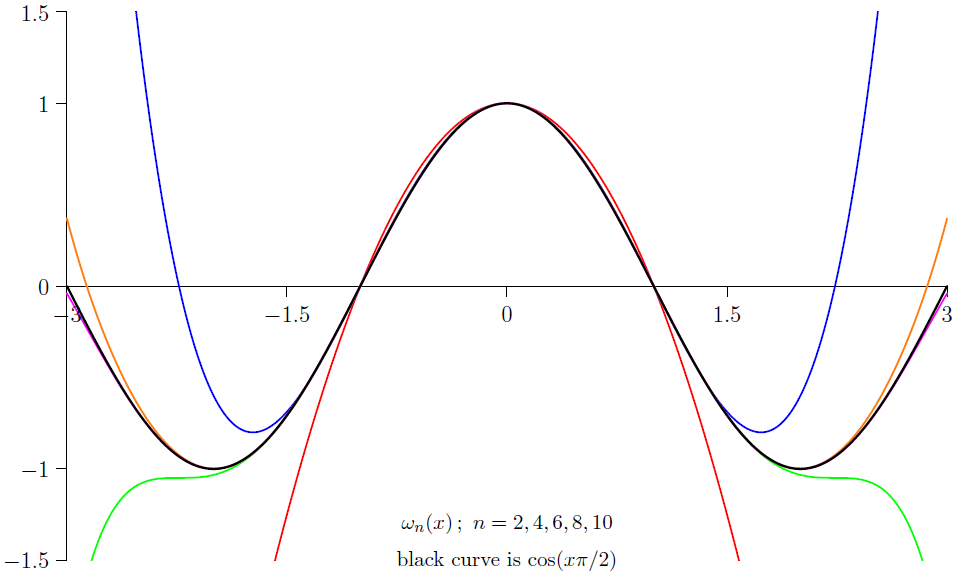Maple Code

The Swiss-Knife polynomials by definition.

SwissKnifePolynomial := proc(n, x) local v, k, pow, chen;
pow := (a,b) -> if a = 0 and b = 0 then 1 else a^b fi;
chen := m -> if irem(m+1,4) = 0 then 0
else 1/((-1)^iquo(m+1,4)*2^iquo(m,2)) fi;
end:

Swiss-Knife polynomials, triangle of coefficients:

for i from 0 to 9 do
p := sort(expand(SwissKnifePolynomial(i,x)));
print(seq(coeff(p,x,i-j),j=0..i)): od:

1
1, 0
1, 0, -1
1, 0, -3, 0
1, 0, -6, 0, 5
1, 0, -10, 0, 25, 0
1, 0, -15, 0, 75, 0, -61
1, 0, -21, 0, 175, 0, -427, 0
1, 0, -28, 0, 350, 0, -1708, 0, 1385
1, 0, -36, 0, 630, 0, -5124, 0, 12465, 0

A representation of the Swiss-Knife polynomials by a single sum.

SKPolynomial := proc(n,x) local k;

for n from 0 to 7 do SKPolynomial(n,x) od;

1
x
x^2 - 1
x^3 - 3 x
x^4 - 6 x^2 + 5
x^5 - 10 x^3 + 25 x
x^6 - 15 x^4 + 75 x^2 - 61
x^7 - 21 x^5 + 175 x^3 - 427 x

An exponential generating function for the SKPolynomials.

G := (x, t) -> 2*exp(x*t)/(exp(t)+exp(-t));

## Sum of alternating powers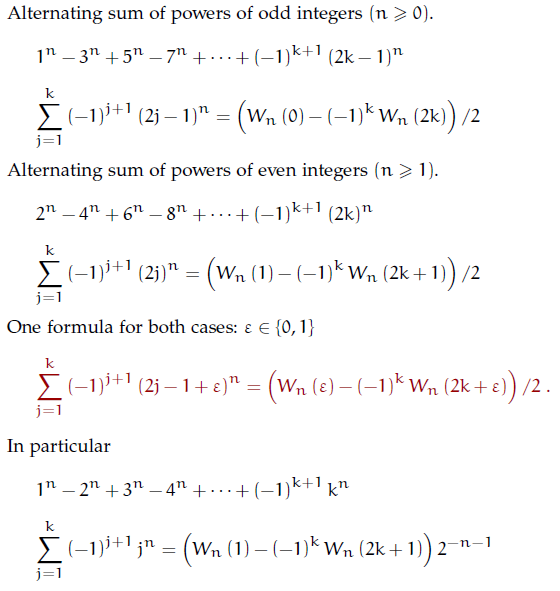Note that all this can be done with integer arithmetic only. So why use some kind of generalized Bernoulli polynomials which are more complicated and use rational arithmetic? Even an evaluation with Euler polynomials has to use rational arithmetic because the coefficients of the Euler polynomials are rational numbers -- in contrast to the coefficients of the Swiss-Knife polynomials which are integers. And... it's just nice to know that it can be done by conceptual simpler polynomials.

It seems much more interesting to ask how much of the functionality the generalized Bernoulli or Euler polynomials provide can be reconstructed in terms of the Swiss-Knife polynomials.

## A surprising connection with the von Mangoldt function

The greatest common divisor of the nonzero coefficients of the decapitated Swiss-Knife polynomials is exp(Lambda(n)), where Lambda(n) is the von Mangoldt function for odd primes [OEIS-A155457].

seq(igcd(coeffs(expand(SwissKnifePolynomial(i,x)-x^i))),i=2..49);

1, 3, 1, 5, 1, 7, 1, 3, 1, 11, 1, 13, 1, 1, 1, 17, 1, 19, 1, 1, 1, 23, 1, 5, 1, 3, 1, 29, 1, 31, 1, 1, 1, 1, 1, 37, 1, 1, 1, 41, 1, 43, 1, 1, 1, 47, 1, 7

## The complementary Swiss-Knife Polynomials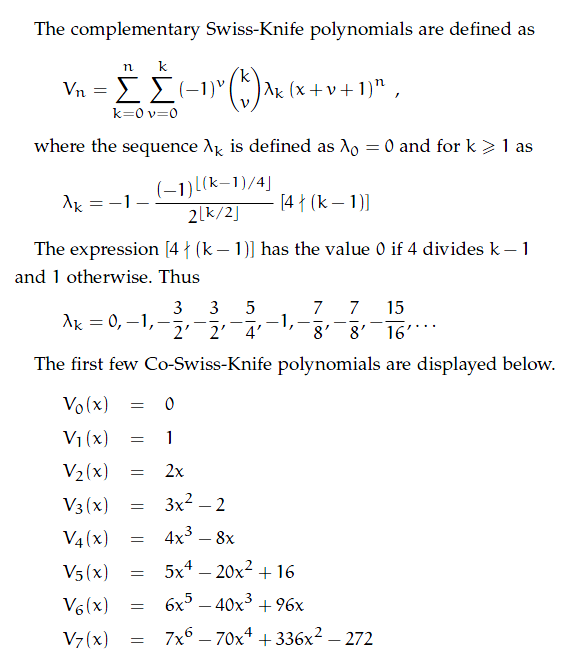The Swiss-Knife polynomials are complementary to the polynomials defined here. Adding both gives polynomials which we will study below.

Maple Code

The Co-Swiss-Knife polynomials by definition.

CoSwissKnifePolynomial := proc(n,x)
local v, k, pow, iver, lambda;
iver := k -> if k mod 4 <> 0 then 1 else 0 fi:
lambda := k -> if(k=0,0,-1-iver(k-1)*(-1)^iquo(k-1,4)/2^iquo(k,2));
pow := (a, b) -> if a = 0 and b = 0 then 1 else a^b fi;
k=0..n) end:

Co-Swiss-Knife polynomials, triangle of coefficients:

for i from 0 to 9 do
p := sort(expand(CoSwissKnifePolynomial(i,x)));
print(seq(coeff(p,x,i-j),j=0..i)): od:

0
0, 1
0, 2, 0
0, 3, 0, -2
0, 4, 0, -8, 0
0, 5, 0, -20, 0, 16
0, 6, 0, -40, 0, 96, 0
0, 7, 0, -70, 0, 336, 0, -272
0, 8, 0, -112, 0, 896, 0, -2176, 0
0, 9, 0, -168, 0, 2016, 0, -9792, 0, 7936

A representation of the Co-Swiss-Knife polynomials by a single sum.

CoSKPolynomial := proc(n,x) local k,pow;
pow := (n,k) -> if(n=0 and k=0,1,n^k);

for n from 0 to 7 do CoSKPolynomial(n,x) od;

0
1
2*x
3*x^2-2
4*x^3-8*x
5*x^4-20*x^2+16
6*x^5-40*x^3+96*x
7*x^6-70*x^4+336*x^2-272

An exponential generating function for the CoSKPolynomials.

G := (x, t) -> exp(x*t)*(exp(2*t)-1)/(exp(2*t)+1);

## A comparison with the Bernoulli and Euler polynomials.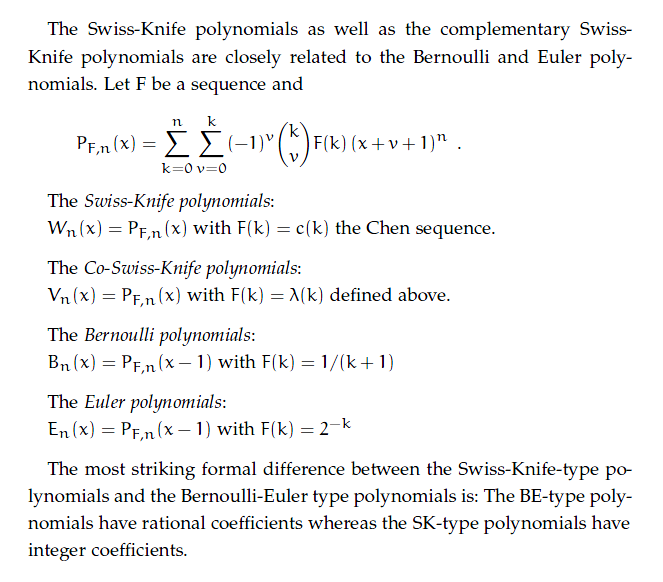## Bernoulli polynomials and harmonic number.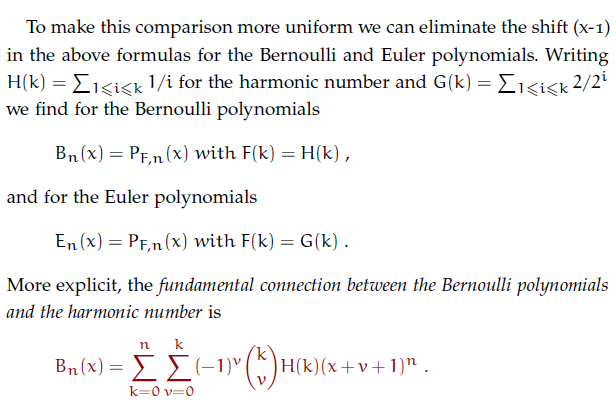##The Swiss-Sword polynomials.

A sword has a blade with two cutting edges. So if we combine the cutting edge of a Swiss-Knife with the cutting edge of a Co-Swiss-Knife we have a Zweihänder in our scabbard and earn twice the pay of a common combinatorialist as a Doppelsöldner of Math.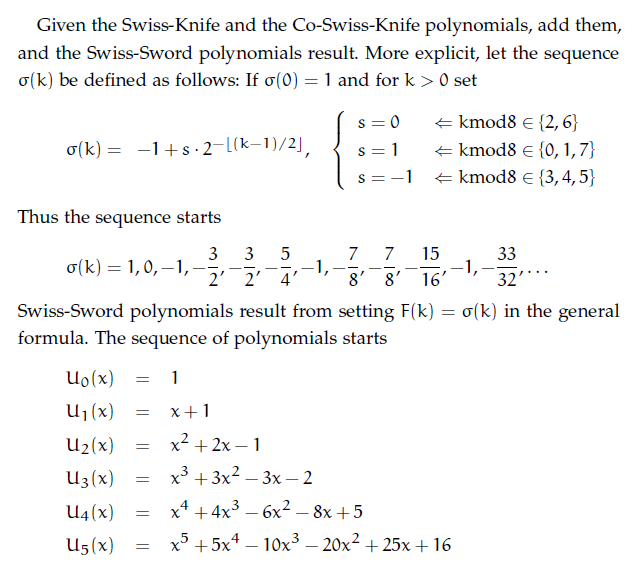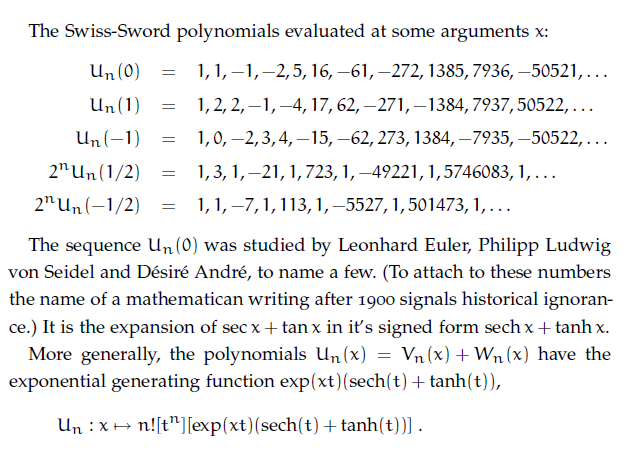• At the Online Encyclopedia of Sequences:

 Swiss-Knife A153641 Co-Swiss-Knife A162660 Swiss-Sword A109449

• The Swiss-Knife polynomials lead in a natural way to an additive decompositions of the Euler, Bernoulli, Genocchi, Springer and tangent numbers.

• The Asymptote code for plotting the scaled Swiss-Knife polynomials.

• A Maple worksheet.

• An article on my OEIS blog: The lost Bernoulli numbers.

• A related article: The Bernoulli Manifesto.